#### You may also like### Pizza Portions

My friends and I love pizza. Can you help us share these pizzas equally?### Tweedle Dum and Tweedle Dee

Two brothers were left some money, amounting to an exact number of pounds, to divide between them. DEE undertook the division. "But your heap is larger than mine!" cried DUM...### Sum Equals Product

The sum of the numbers 4 and 1 [1/3] is the same as the product of 4 and 1 [1/3]; that is to say 4 + 1 [1/3] = 4 � 1 [1/3]. What other numbers have the sum equal to the product and can this be so for any whole numbers?

# Rod Fractions

##### Age 7 to 14 Challenge Level:

Pablo from International School of Brussels in Belgium and students from Holmgate Primary School found the fractions that some rods are of each other. This is Pablo's work: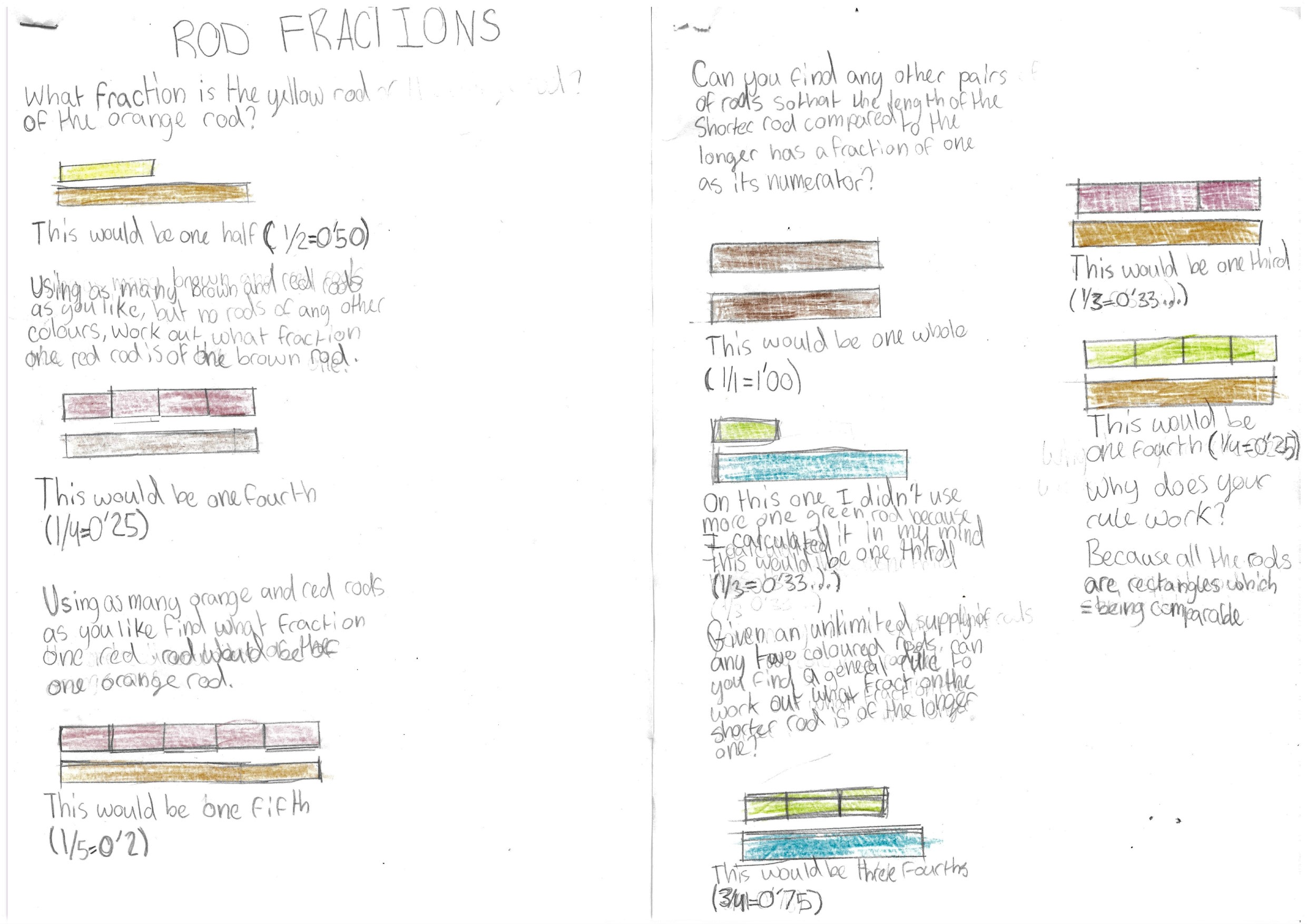... and this is Holmgate Primary School's work: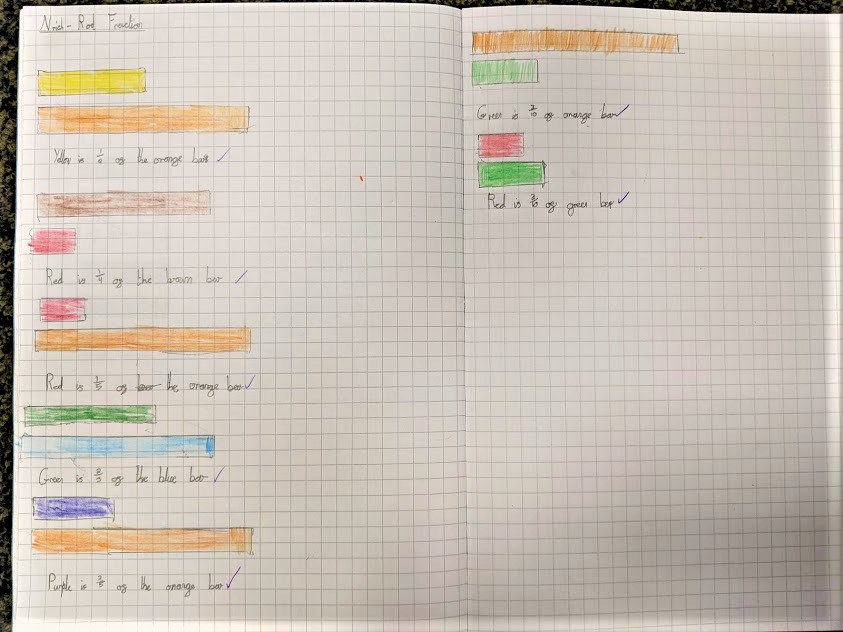Adithya from PSBB KK Nagar Chennai in India made a list of pairs of rods where the length of the shorter rod compared with the longer rod is a fraction with 1 as its numerator:

(Red, Dark Green)
(Red, Light Green)
(Light Green,Dark Green)
(Light Green,Blue)
(Pink,Brown)
(White, Any Colour) 9 possibilities for 10 rods

Keshav from Colchester Royal Grammar School in the UK used a trick to work out how long the rods are compared to each other:

The trick to understanding how the different rods are linked to each other is by looking at their order on the right of the interactivity. At the top is the white rod, and at the bottom is the orange rod. To find the fraction that two rods make - or the number of times the smaller rod is needed to equal the size of the larger one - you need to know where the colour is in the column.

For example, the red rod is second in the column. The pink rod is fourth. If we put these into a fraction, we get $\frac24$, which equals $\frac12$. And, using the interactivity, we find that we do indeed need 2 reds to fill a pink rod. Another example is the green rod and the blue rod. The green is 3rd, and the blue is 9th. Writing this as a fraction, we get $\frac39 = \frac13,$ and we again find that we need 3 green rods to equal the length of a blue rod.

Scout from James Allen Girls School in the UK, and Adithya, both sent in images which show why Keshav's method works. Here is Adithya's image: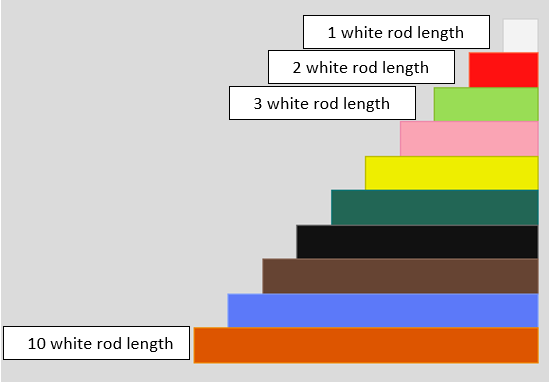... and here is Scout's: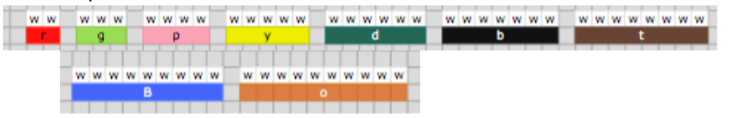Given an unlimited supply of any two differently coloured rods, can you find a general rule to work out what fraction the shorter rod is of the longer one?

Carlos V from Kings College Alicante in Spain and Mahdi from Mahatma Gandhi International School in India both described this general method. This is Carlos' work:

First, you place a rod of one colour over the other rod. Then, continue adding rods until the length of the smaller rods is greater or equal to the larger rod.

If the length of the smaller rods is larger, add another large rod and continue adding smaller rods until they are equal. When it is equal, count how many smaller rods there are and then divide the amount of larger ones there are by the amount of smaller rods. That is the size of the smaller rod compared to the larger rod.

If you want to find the size of the larger rod compared to the smaller rod, divide the amount of smaller ones by the amount of larger rods.

Mahdi also sent in this method, which works if you know the length of the rods (you could measure them using white rods):

For any two rods of length ‘$x$’ and ’$y$’, when you place $y$ number of rods of length $x$ straight in a line, it will make the same length as placing $x$ number of rods of length $y$ in a line. To make this a bit more clear, let us go through an example:

You have rods of length 4 and 7. So with the two rods of 4 and 7, place 7 such pink (4 unit) rods and 4 of the black (7 units).We observe that the lengths are equal. Using this, we can calculate the ratio of both lengths easily. Intuitively, we can see why the statement in the first line is true because $y$ number of rods of length $x$ makes a total length of $xy$ and vice versa.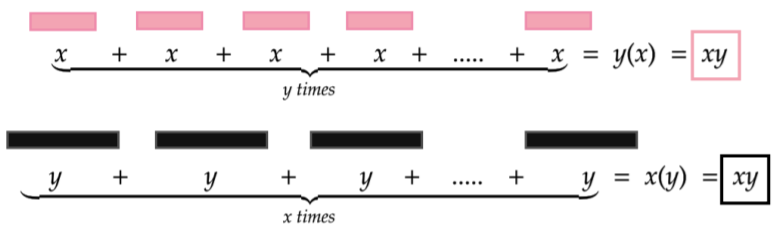Aditya from Bishop Vesey's Grammar School in the UK also measured the rods and described this neat method which uses the Lowest Common Multiple (LCM):

The way one can work out what fraction the shorter rod is of the longer one is by essentially finding an LCM and simplifying the ratio into an $1:n$ form.

Firstly, to find the lowest common multiple between the two, you will need some sort of unit or measure to calculate with. I used the interactivity's grid squares and measured each square as $1$ unit. Using this, you can find an LCM between the lengths of the 2 rods. Using this, I then formed a ratio. For example, if we were to use the yellow and dark green rods, they both have a length of $5$ and $6$ units respectively and have an LCM of $30$. This signifies that if the two types of rods were placed consecutively under they both met at a column, there would be a total length of $30$.

This means that there are ($30\div5$) $6$ yellow rods and ($30\div6$) $5$ dark green rods. This allows us to form a ratio that there are $5$ dark green rods to every $6$ yellow rods. If put in the form of $1:n$, there would be $1$ green rod to every $1.2$ yellow rod - $1:1.2$